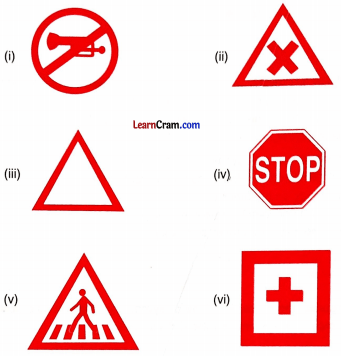# DAV Class 7 Maths Chapter 13 Worksheet 5 Solutions

The DAV Class 7 Maths Book Solutions and DAV Class 7 Maths Chapter 13 Worksheet 5 Solutions of Symmetry offer comprehensive answers to textbook questions.

## DAV Class 7 Maths Ch 13 WS 5 Solutions

Question 1.
In the following figures, the mirror line (line of symmetry) is given as a dotted line. Complete each figure (you may use a mirror on the dotted line).### DAV Class 7 Maths Chapter 13 Value Based Questions

Question 1.
Neha went to the market with her mother. There was a lot of traffic on the road. Neha saw a few traffic signs on the road side.(i) Which of these signs have lines of symmetry? Write the number of lines of symmetry for each.
(ii) Find out the meaning of each of these signs. Why is it important to obey traffic rides?